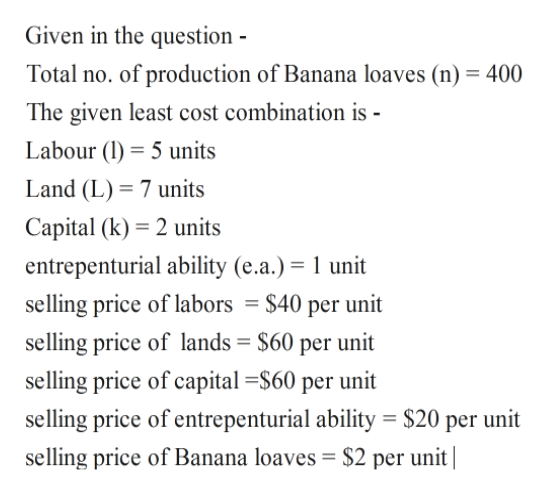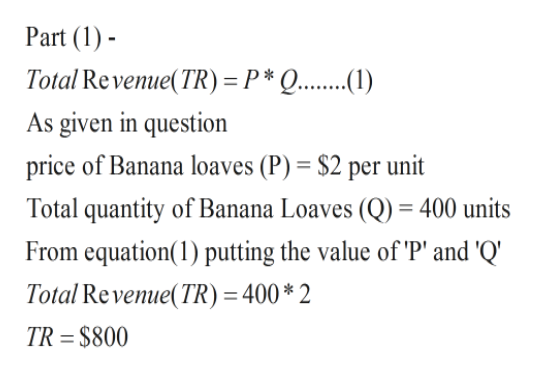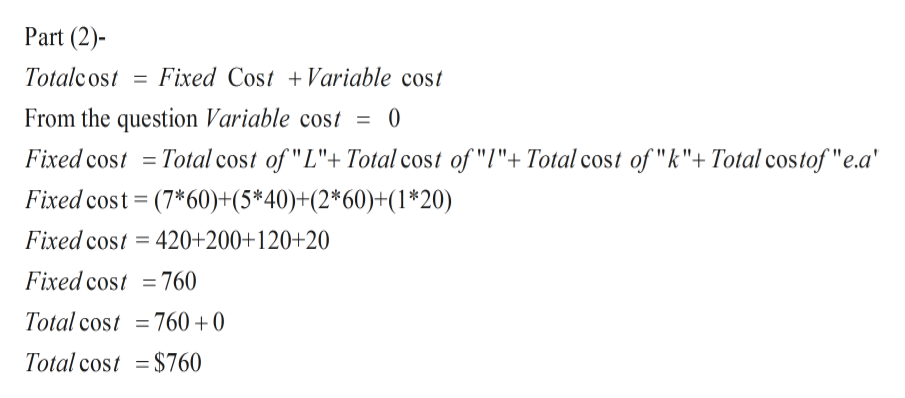With current technology, suppose a firm is producing 400 loaves of banana bread daily. Also assume that the least-cost combination of resources in producing those loaves is 5 units of labor, 7 units of land, 2 units of capital, and 1 unit of entrepreneurial ability, selling at prices of \$ 40, \$60, \$60, and \$20, respectively. Assume the firm can sell these 400 loaves at \$ 2 per unit. 1). What is its total revenue?2). What is its total cost?3).  What is the firm's profit or loss? The firm generates an economic ( choose one -a) loss, b) profit ) of...? 4). Will it continue to produce banana bread? ( Yes or No) 5). If this firm's situation is typical for the other makers of banana bread, will resources flow toward or away from this bakery good?

Question

With current technology, suppose a firm is producing 400 loaves of banana bread daily. Also assume that the least-cost combination of resources in producing those loaves is 5 units of labor, 7 units of land, 2 units of capital, and 1 unit of entrepreneurial ability, selling at prices of \$ 40, \$60, \$60, and \$20, respectively. Assume the firm can sell these 400 loaves at \$ 2 per unit.

1). What is its total revenue?

2). What is its total cost?

3).  What is the firm's profit or loss? The firm generates an economic ( choose one -a) loss, b) profit ) of...?

4). Will it continue to produce banana bread? ( Yes or No)

5). If this firm's situation is typical for the other makers of banana bread, will resources flow toward or away from this bakery good?

Step 1

Solution- Dear student Thank you for submitting your question. Since we only answer up to three sub parts we will answer the first three. Please resubmit the question and specify other sub –parts (up to 3) you’d like to answered.help_outlineImage TranscriptioncloseGiven in the question Total no. of production of Banana loaves (n) 400 The given least cost combination is - Labour (5 units Land (L) 7 units Capital (k) 2 units entrepenturial ability (e.a.) 1 unit selling price of labors = \$40 per unit selling price of lands \$60 per unit selling price of capital S60 per unit selling price of entrepenturial ability \$20 per unit selling price of Banana loaves = \$2 per unit| fullscreen
Step 2help_outlineImage TranscriptionclosePart (1 Total Revenue(TR) =P* Q... As given in question price of Banana loaves (P) = \$2 per unit Total quantity of Banana Loaves (Q) = 400 units From equation(1) putting the value of 'P' and 'Q' Total Revenue(TR) = 400* 2 TR = \$800 fullscreen
Step 3help_outlineImage TranscriptionclosePart (2) Totalcost Fixed Cost Variable cost From the question Variable cost 0 Total cost of "L"+ Total cost of "l"+ Total cost of "k"+Total costof "e.a Fixed cost Fixed cost (7*60)+(5*40)+(2*60)+(1*20) Fixed cost = 420+200+120+20 Fixed cost =760 Total cost =760 +0 Total cost = \$760 fullscreen

Want to see the full answer?

See Solution

Want to see this answer and more?

Our solutions are written by experts, many with advanced degrees, and available 24/7

See Solution
Tagged in